Last Updated: July 22, 2021

# How to build an AI algorithm that solves Sudoku puzzles

The broad approach of Artificial Intelligence (AI) is to replicate the rational abilities of humans in a computational environment. One of the best ways to evaluate the capabilities of an AI is to see if they can beat humans at playing games:

• In the 1990’s, Deep Blue defeated a reigning world Chess champion for the first time.
• In the 2010’s, AlphaGo became the first computer to defeat humans at the game of Go. Currently, AlphaZero is considered to be the best Go player in the world.

Given the complexities of Chess and Go, Sudoku should be a piece of cake. Of course, in some cases, the cake is a lie. While AI can use reason to select winning strategies, a bit of human ingenuity and coding logic (using a technique called backtracking) can work just as well.

1. Create a Python environment that contains all the packages we’ll need for the task
2. Define our approach: Brute Force vs Machine Learning
3. Import Kaggle’s Sudoku puzzle dataset
4. Test out the Brute Force method
5. Build an algorithm to solve Sudoku puzzles
6. Draw some conclusions about whether AI solves Sudoku more efficiently than humans

All of the code used in this article can be found on my GitLab repository. All set? Let’s go.

## 1–Before you start: Install the Sudoku Solver Python Environment

To follow along with the code in this article, you can download and install our pre-built Sudoku Solver environment, which contains a version of Python 3.9 and the packages used in this post.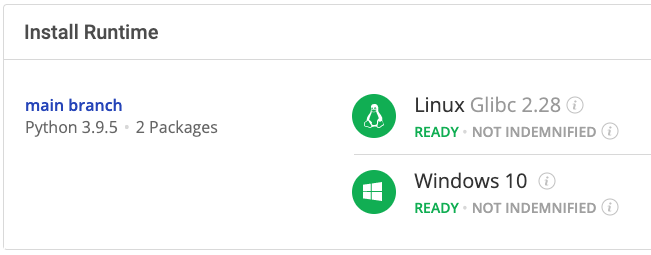Or you could also use our State tool to install this runtime environment.

For Windows users, run the following at a CMD prompt to automatically download and install our CLI, the State Tool along with the Sudoku Solver into a virtual environment:

`powershell -Command "& \$([scriptblock]::Create((New-Object Net.WebClient).DownloadString('https://platform.activestate.com/dl/cli/install.ps1'))) -activate-default Pizza-Team/SudokuSolver"`

For Linux users, run the following to automatically download and install our CLI, the State Tool along with the Sudoku Solver into a virtual environment:

`sh <(curl -q https://platform.activestate.com/dl/cli/install.sh) --activate-default Pizza-Team/SudokuSolver`

## 2–Ways to Solve Sudoku with Python

A Sudoku puzzle consists of a 9 by 9 grid, where each cell contains a number between 1 and 9. The 9 by 9 grid is divided into 9 subgrids defined by the intersection of the first, middle, and last 3 columns and the first, middle, and last 3 rows. The solution to the puzzle must satisfy the following conditions:

• Each row must contain 1 through 9, without repetition.
• Each column must contain 1 through 9, without repetition.
• Each subgrid must contain 1 through 9, without repetition.

Of course, the puzzle is given with a number of the cells empty, and the goal is to fill in the empty cells until the conditions are satisfied. For example: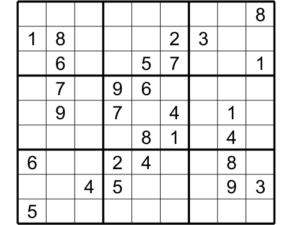### Approaching the Problem

In the case of Deep Blue, the program was designed to evaluate the relative risk of every possible position for each Chess piece at each turn. This relies heavily on brute force computing, which requires either a lot of time, a lot of computation power, or both.

The complexity of Chess can be quantified by calculating the total number of board positions, which equals roughly 1047 possibilities. On the other hand, the game of Go has approximately 10170 different configurations. This makes it infeasible to use a brute force algorithm to play Go, so AlphaGo uses a combination of Machine Learning (ML) and tree algorithms to make in-game decisions.

The complexity of Sudoku depends on the number of empty cells in the grid. If 41 of the 81 cells are empty and each cell can take values from 1 to 9, there are 941 possible configurations. This makes Sudoku similar in complexity to Chess, where brute force algorithms are feasible to implement. This will be our approach to solving Sudoku puzzles using Python.

## 3–Sudoku Dataset

Fortunately, Kaggle has a publicly available dataset of 9 million Sudoku puzzles and their solutions, which can be found here. After downloading the .csv file, we can import the puzzles by running:

`import numpy as npimport pandas as pdimport timeimport itertoolssudoku_df = pd.DataFrame(pd.read_csv('sudoku.csv', nrows = 10**2))`

I only imported the first 100 puzzles, but feel free to import more as needed. Each row of the dataframe contains a puzzle and the corresponding solution. They should look like this:To visualize each puzzle in the standard 9 by 9 grid instead of a string of 81 characters, we can write a function to reshape it:

`def shape(sudoku_df):    for n in range(sudoku_df.shape):        sudoku_df.iloc[n,0] = np.reshape(list(sudoku_df.puzzle.values[n]),(9,9)).astype(int)        sudoku_df.iloc[n,1] = np.reshape(list(sudoku_df.solution.values[n]),(9,9)).astype(int)    return sudoku_df`

And to look at the first puzzle:

`sudoku_df = shape(sudoku_df)sudoku_df.iloc[0,0]`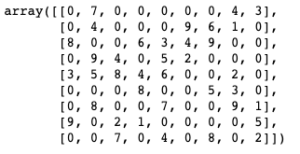The zeroes represent the empty cells that we need to fill. The solution looks like this:

`sudoku_df.iloc[0,1]`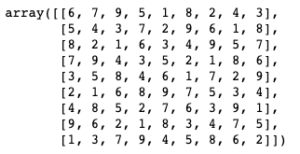Before we explain how to solve the puzzle, we should implement the three Sudoku conditions to verify that any proposed solution is in fact a solution. This can be done with the following function:

`def checkPuzzle(sudoku_puzzle):    checkRow = all([all([x in sudoku_puzzle[nrow,:] for x in range(1,10)]) for nrow in range(9)])    checkCol = all([all([x in sudoku_puzzle[:,ncol] for x in range(1,10)]) for ncol in range(9)])    checkUpperLeft = all([x in sudoku_puzzle[0:3,0:3] for x in range(1,10)])    checkUpperMid = all([x in sudoku_puzzle[0:3,3:6] for x in range(1,10)])    checkUpperRight = all([x in sudoku_puzzle[0:3,6:9] for x in range(1,10)])    checkMidLeft = all([x in sudoku_puzzle[3:6,0:3] for x in range(1,10)])    checkMidMid = all([x in sudoku_puzzle[3:6,3:6] for x in range(1,10)])    checkMidRight = all([x in sudoku_puzzle[3:6,6:9] for x in range(1,10)])    checkLowerLeft = all([x in sudoku_puzzle[6:9,0:3] for x in range(1,10)])    checkLowerMid = all([x in sudoku_puzzle[6:9,3:6] for x in range(1,10)])    checkLowerRight = all([x in sudoku_puzzle[6:9,6:9] for x in range(1,10)])    solved = all([checkRow,checkCol,checkUpperLeft,checkUpperMid,checkUpperRight,                  checkMidLeft,checkMidMid,checkMidRight,checkLowerLeft,checkLowerMid,checkLowerRight])    if solved:        for line in sudoku_puzzle:            print(*line)    return solved`

For each problem’s solution, we check that:

• Each row contains all the numbers 1 through 9
• Each column contains all the numbers 1 through 9
• Each subgrid contains all the numbers 1 through 9

If they all check out, the function outputs True; if not, it outputs False. Feel free to try it out with the solution to the first puzzle.

## 4–Sudoku Solving with Brute Force

To build an algorithm to solve any Sudoku puzzle, we really only need to solve a single puzzle. We can then generalize each function such that any puzzle can be the input. Let’s take the puzzle from above, which has 44 empty cells:

`len(np.where(sudoku_df.iloc[0,0] == 0))`

If we assume that each cell can be 9 values, this means that there are around 1041 possible solutions:

`"{:.2e}".format(9**len(np.where(sudoku_df.iloc[0,0] == 0)))`

However, this does not take the values of the cells that are already filled in into consideration. Using these values (as well as our initial three conditions – the rules of Sudoku), we can figure out which values are allowed in each cell:

`def determineValues(sudoku_puzzle):    puzzle_values = list()    for r in range(9):        for c in range(9):            if sudoku_puzzle[r,c] == 0:                cell_values = np.array(range(1,10))                cell_values = np.setdiff1d(cell_values,sudoku_puzzle[r,:][np.where(sudoku_puzzle[r,:] != 0)]).tolist()                cell_values = np.setdiff1d(cell_values,sudoku_puzzle[:,c][np.where(sudoku_puzzle[:,c] != 0)]).tolist()            else:                cell_values = [sudoku_puzzle[r,c]]            puzzle_values.append(cell_values)    return puzzle_values`

In the above code, the function:

• Scans through each cell and determines the values that are already in the cell’s row, column, and subgrid.
• Removes known values from our list of values from 1 through 9 (if the cell is already filled, the only possible value is the one that is already given).
• The output is a list of the possible values of each cell.

We can now run the function on the first puzzle as follows:

`puzzle_values = determineValues(sudoku_df.iloc[0,0])puzzle_values`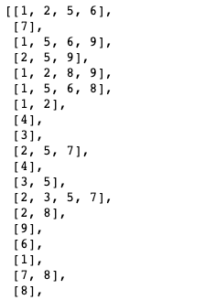So, going left to right and top to bottom:

• The first cell has possible values of 1, 2, 5, or 6.
• The second cell already has a 7.
• The third cell could have a 1, 5, 6, or 9.

And so on. The list should have a total length of 81 lines, which corresponds to the total number of cells (9×9). Now that we know the possible values of each cell, this constrains the complexity of the puzzle. The number of possibilities is now:

`combinations = 1for i in range(81):    combinations = combinations*len(puzzle_values[i])"{:.2e}".format(combinations) '1.25e+19'`

That’s still quite high, but much better than before. It’s possible to write a function that generates all 1.25e19 of these 9 by 9 matrices and then uses our checkPuzzle function to scan through each one until the solution is found. Despite the simplicity of such an exercise, the process would be computationally intensive. To see how feasible it is, we can include a timer and only scan through a fraction of the possibilities to determine the approximate total time. That function would look something like this:

`def bruteForce_check(puzzle_values):    first = np.array(np.meshgrid(*puzzle_values[0:27])).T.reshape(-1,3,9)    second = np.array(np.meshgrid(*puzzle_values[27:54])).T.reshape(-1,3,9)    third = np.array(np.meshgrid(*puzzle_values[54:])).T.reshape(-1,3,9)    start_time = time.time()    for i in range(first.shape):        for j in range(second.shape):            for k in range(third.shape):                potential_solution = np.concatenate((first[i],second[j], third[k]))                solution = checkPuzzle(potential_solution)                iterations = 10**4                if (i+1)*(j+1)*(k+1) == iterations:                    current_time = time.time()                    run_time = current_time - start_time                    print('Projected Number of Days: ')                    print("{:.2e}".format(combinations*(run_time/iterations)/(24*3600)))                    break                else:                    continue            break        break`

Note that I combined the first three rows, the middle three rows, and last three rows in this way because numpy does not allow arrays with 81 dimensions. I stopped the function after 104 iterations and projected the iteration rate over the total number of potential solutions:

`bruteForce_check(puzzle_values)Projected Number of days:1.50e+11`

That’s quite a long time! Clearly this approach is not ideal. Instead, we will implement something called Backtracking

## 5–Sudoku Solving with Backtracking

Our brute force approach involved determining the possible values of each cell, generating all possible 9 by 9 grids, and scanning through each one. A better approach would be to pick a cell to start with, and then:

1. Insert a number from our list, and check the validity conditions.
1. If the conditions are valid, move to a new cell.
2. If the conditions are invalid, pick the next number in the list.
2. Repeat this process until either all values in the cell’s list have failed the validity check or all cells have been filled.
1. If the cell’s values have been exhausted, move to the previous cell and pick a different value, then repeat.

In this way, we check the validity of each cell as we construct the solution and reject anytime a value doesn’t fit. To implement this in Python, we first need a function that efficiently checks the subgrid corresponding to the current cell (this will come in handy later):

`def checkGrids(r,c,sudoku_puzzle,n):    if r < 3:        if c < 3:            subgrid = n in sudoku_puzzle[0:3,0:3]        elif c < 6:            subgrid = n in sudoku_puzzle[0:3,3:6]         else:            subgrid = n in sudoku_puzzle[0:3,6:9]    elif r < 6:        if c < 3:            subgrid = n in sudoku_puzzle[3:6,0:3]        elif c < 6:            subgrid = n in sudoku_puzzle[3:6,3:6]        else:            subgrid = n in sudoku_puzzle[3:6,6:9]            else:        if c < 3:            subgrid = n in sudoku_puzzle[6:9,0:3]        elif c < 6:            subgrid = n in sudoku_puzzle[6:9,3:6]        else:            subgrid = n in sudoku_puzzle[6:9,6:9]      return subgrid`

In theory, the pathway that the function takes through the grid and the order of each cell’s values will both influence the total time to find a solution. However, there is no way to know beforehand which pathway or order is best. Thus, we will scan through the empty cells from left to right and top to bottom, and we’ll also scan the values from smallest to greatest.

I will define a few utility variables to keep track of:

• Current cell (count)
• Solution status of the puzzle (solution)
• Dictionary of each cell’s present and past value index (num and dic

Once the last empty cell is filled with a valid value, the whole matrix is double-checked with the checkPuzzle function:

`def solve(sudoku_puzzle,puzzle_values):    count = 0    solution = False    rows = np.array(np.where(sudoku_puzzle == 0))    cols = np.array(np.where(sudoku_puzzle == 0))    dic = dict(zip(list(range(len(rows))), np.zeros(len(rows),dtype = int).tolist()))    while solution == False:        if count >= len(rows):            solution = checkPuzzle(sudoku_puzzle)            break        r = rows[count]        c = cols[count]        len_num = len(np.array(puzzle_values).reshape(9,9)[r,c])        num = dic[count]        while num < len_num:            cell = np.array(puzzle_values).reshape(9,9)[r,c][num]            checkRow = cell in sudoku_puzzle[r,:]            if checkRow:                num += 1                continue            checkCol = cell in sudoku_puzzle[:,c]            if checkCol:                num += 1                continue            checkGrid = checkGrids(r,c,sudoku_puzzle,cell)            if checkGrid:                num += 1                continue            dic[count] = num            count += 1            sudoku_puzzle[r,c] = cell            break        else:            sudoku_puzzle[r,c] = 0             dic[count] = 0            count -= 1`

The above function:

1. Checks the validity of each row, column, and subgrid:
1. If it fails, the next value in puzzle_value[r,c] is chosen
2. If it passes, the value is assigned to the cell and we move on to the next cell (and count increases by 1)
2. If none of the values for a given cell pass (i.e. else is executed):
1. The cell value is reset to 0
2. The loop through the potential values is reset
3. We move back to the previous cell (and count decreases by one).

To run the code on the first puzzle, we run:

`solve(sudoku_df.iloc[0,0],puzzle_values)`That’s much quicker than the previous brute force method!

## Conclusions: Python Solves Sudoku Faster than Humans

While AI and Machine Learning are getting a lot of love from the Python community, they’re not always the best way to solve a problem. On the other hand, without access to a supercomputer, brute force solutions can be a non-starter.

Solving Sudoku lies somewhere in between: too complex to solve by brute force (humans are far more efficient), but not if we’re clever about how we go about it. With the addition of some backtracking logic, we can test out values for each cell, and solve the puzzle in a fraction of the time, often quicker than a human could. There’s nothing special about the solution, which uses the power of silicon-based logic (plus numpy & pandas) to outperform human logic. For another approach, see solving Sudoku with AI

Feel free to try out both approaches with any of the other 9 million puzzles in the Kaggle dataset.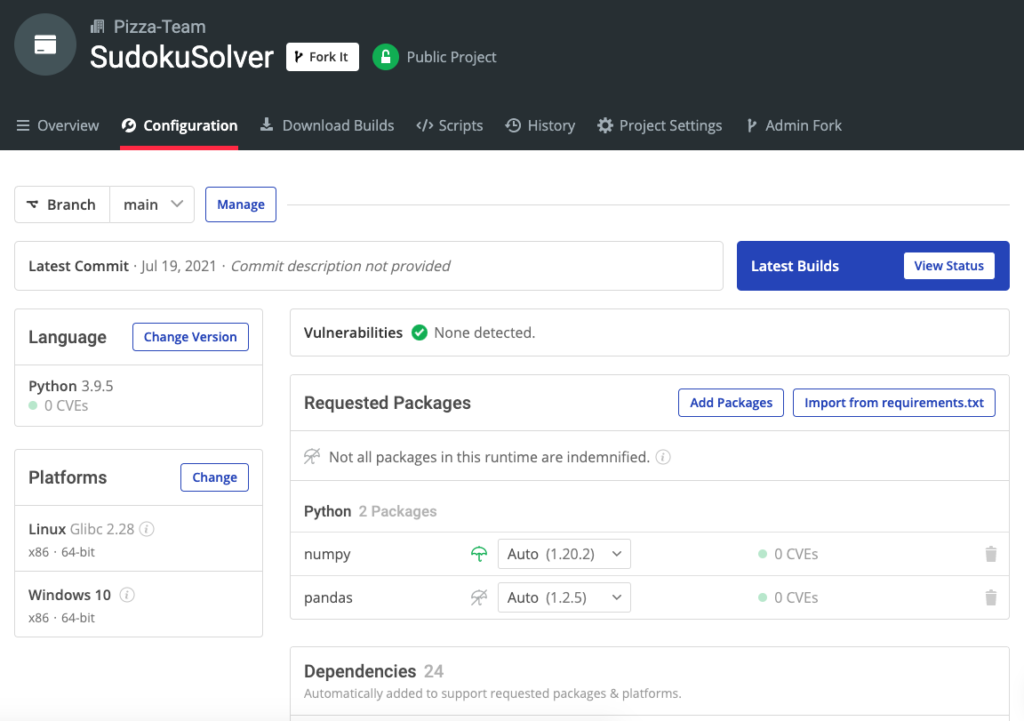With the ActiveState Platform, you can create your Python environment in minutes, just like the one we built for this project. Try it out for yourself or learn more about how it helps Python developers be more productive.